# How To Train ML Models With Mislabeled Data

Rule n° 1 in data science: Garbage In = Garbage Out.

# A brief introduction to the dataset: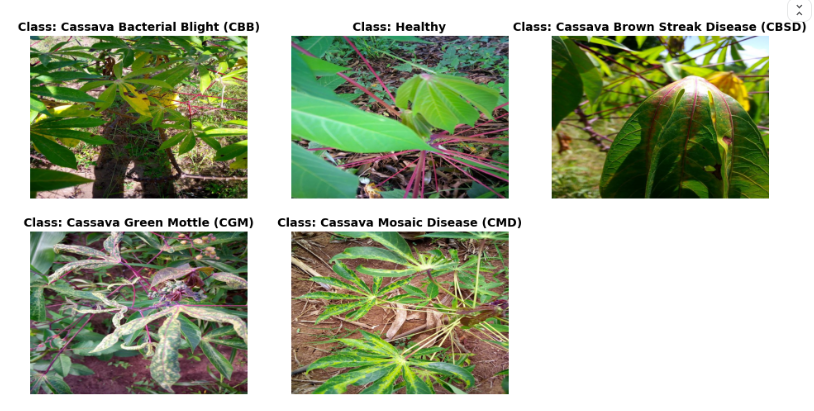Figure 1. Cassava plant classes 4 diseases and one healthy class: CBB, Healthy, CBSD, CGM and CMD [Image by Author]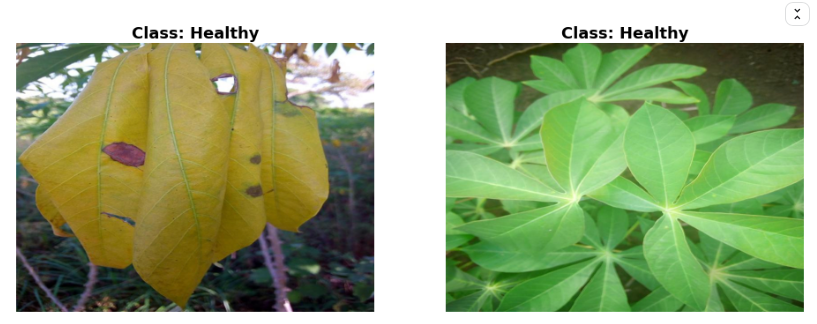Figure 2. An example of a mislabeled image (left) and a correctly labeled image (right) [Image By Author]

# 1- Bi-Tempered loss function: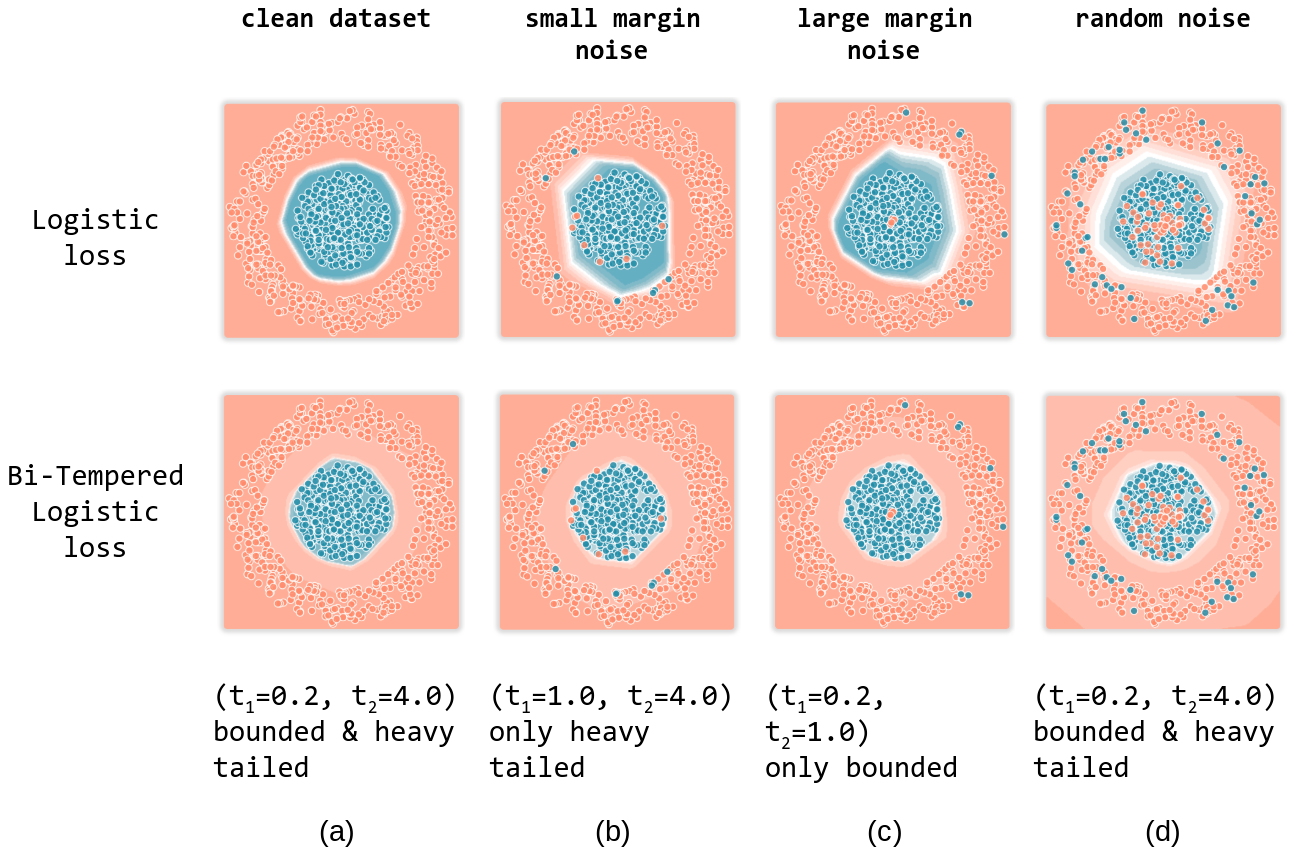Figure 3. Difference in decision boundaries between logistic loss (cross-entropy loss) and Bi-Tempered loss Source: https://ai.googleblog.com/2019/08/bi-tempered-logistic-loss-for-training.html
• With small margin noise: The noise stretched the decision boundary in a heavy tailed form. This was solved with the Bi-Tempered loss by tuning the t2 parameter from t2=1 to t2=4.
• With large margin noise: The large noise stretched the decision boundary in a bounded way, covering more surface than the heavy tail in the case of small margin noise. The Bi-Tempered loss solved this by tuning the t1 parameter from t1=1 to t1=0.2.
• With random noise: Here, we can see both heavy tails and bounded decision boundaries, so both t1 and t2 are adjusted in the Bi-Tempered loss.
`#Import packagefrom mlxtend.plotting import plot_decision_regions# Plot decision boundaryplot_decision_regions(x=x_test, y=y_test, clf=model, legend=2)plt.show()`

# 2- Self Distillation:

Self Distillation: You train your model and then you retrain it using itself as a teacher.

• 1- Split your dataset to k folds cross-validation.
• 2- Train model 1 to predict the out of folds predictions.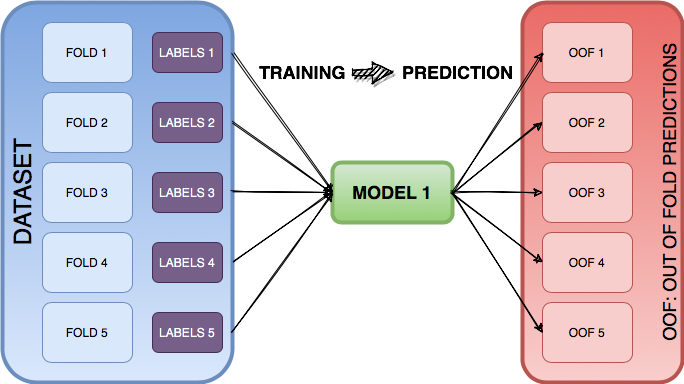Figure 4. The dataset is split to 5 folds cross-validation. Model 1 predicts out of fold predictions. [Image by Author]
• 3- After saving the out of folds predictions predicted by our model, we load them and blend them with the original labels. The blending coefficients are tunable, the original labels should have a higher coefficient.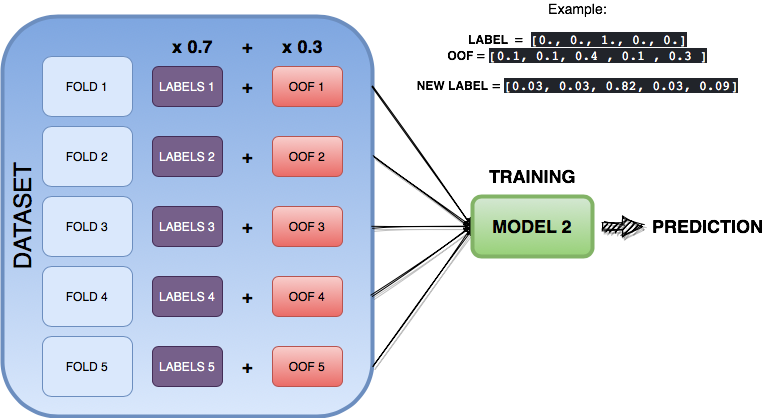Figure 5. Model 2 uses out of fold predicitons (OOF) from Model 1 to improve its performance. [Image by Author]
• In this particular example we have a multiclass classification with 5 classes [0,1,2,3,4].
• The labels are one hot encoded. Class 2 is represented as [0,0,1,0,0].
• Model 1 predicted the class 2 correctly: [0.1, 0.1 ,0.4 ,0.1 ,0.3]. Giving it a probability of 0.4, higher than the other classes. But, it also gave class 4 a high probability of 0.3.
• Model 2 will use this information to improve its predictions.

# 3- Ensemble learning: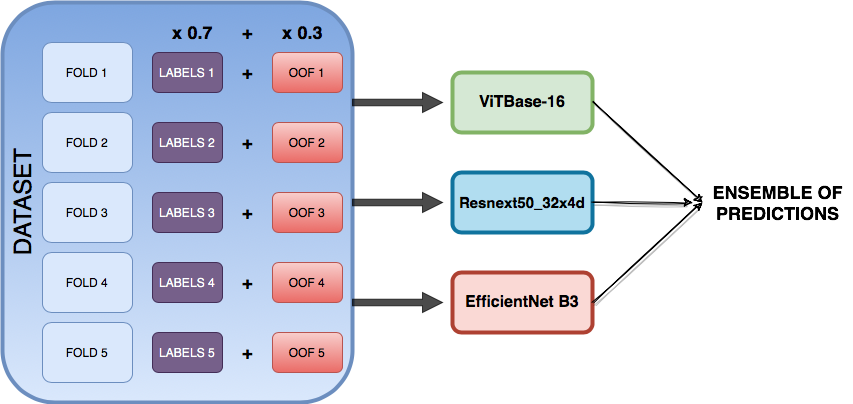Figure 6. Ensemble of 3 different models: VitBase-16, resnext50_32x4d and EfficientNet B3. [Image by Author]
• The Bi-Tempered loss function and tuning its parameters t1 and t2 correctly.
• Self Distillation: Train your model and retrain it again using itself as a teacher.
• Ensemble learning: Ensemble the predictions of different models.

THE END

--

--